## Wednesday, December 3, 2014

### Math lesson 9 areas square rectangle triangle

Areas are the opposite to perimeters. Areas are whats inside the space.

This is also another good strategy for a architect.

To work out an area of a square you have to do side squared - thats how long the side is and times it by itself.

For a rectangle you have to times the width by the hight so its w Times h.

Here are some of my practices

Square - side = 4  Area = 16
side = 10  Area = 40
side = 24  Area = 96

Rectangle
width = 2  hight = 4  Area = 8
width = 6  hight = 10  Area = 60
width = 12  hight = 30  Area = 360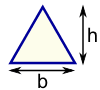Triangle Area = ½ × b × h b = base h = vertical heightSquare Area = a2 a = length of side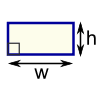Rectangle Area = w × h w = width h = heightParallelogram Area = b × h b = base h = vertical height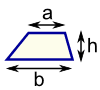Trapezoid (US) Trapezium (UK) Area = ½(a+b) × h h = vertical height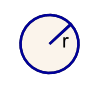Circle  Area = π × r2 Circumference = 2 × π × r r = radius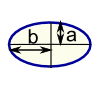Ellipse Area = πabSector Area = ½ × r2 × θ  r = radius θ = angle in radians
 Note: h is at right angles to b: